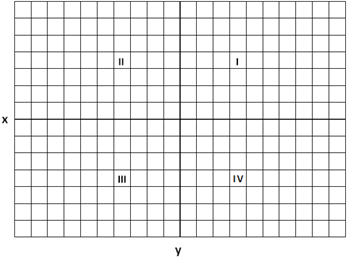# GMAT : Coordinate Geometry Quiz

Quiz
*Theme/Title: Coordinate Geometry
* Description/Instructions
One topic that is generally represented by a few questions on the GMAT is coordinate geometry. This system allows for graphical representation of lines and shapes. In the coordinate system each point is identified in the following way (x, y) where x is the horizontal value and y is represents the vertical value. The coordinate system is divided into four quadrants as shownSlope of a line can be found with the following equation: y = mx + b where m is the slope and b is the y-intercept.
The distance between two points can be found with the following equation:
$d=\sqrt{{\left({y}_{2}-{y}_{1}\right)}^{2}+{\left({x}_{2}-{x}_{1}\right)}^{2}}$

Group: GMAT GMAT Quizzes
Topic: GMAT